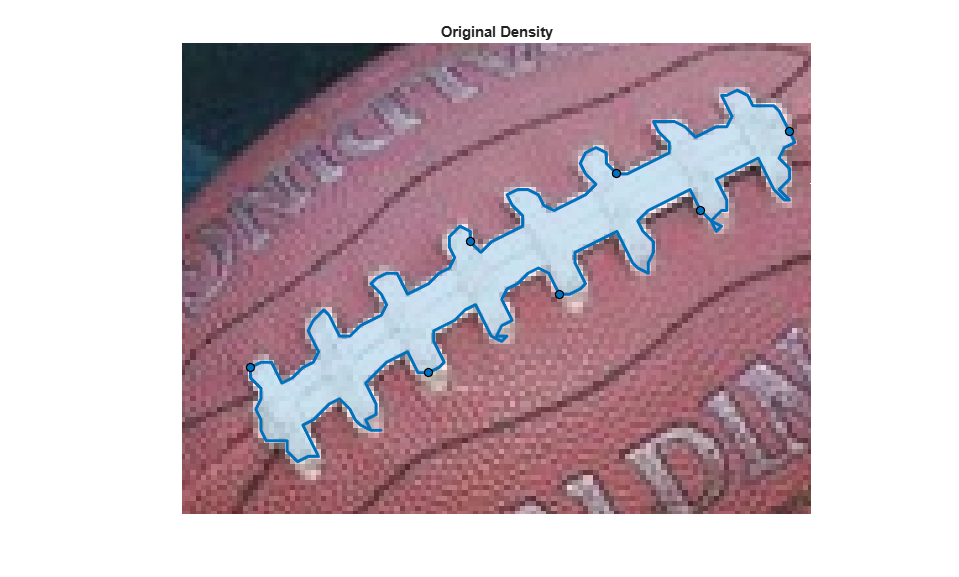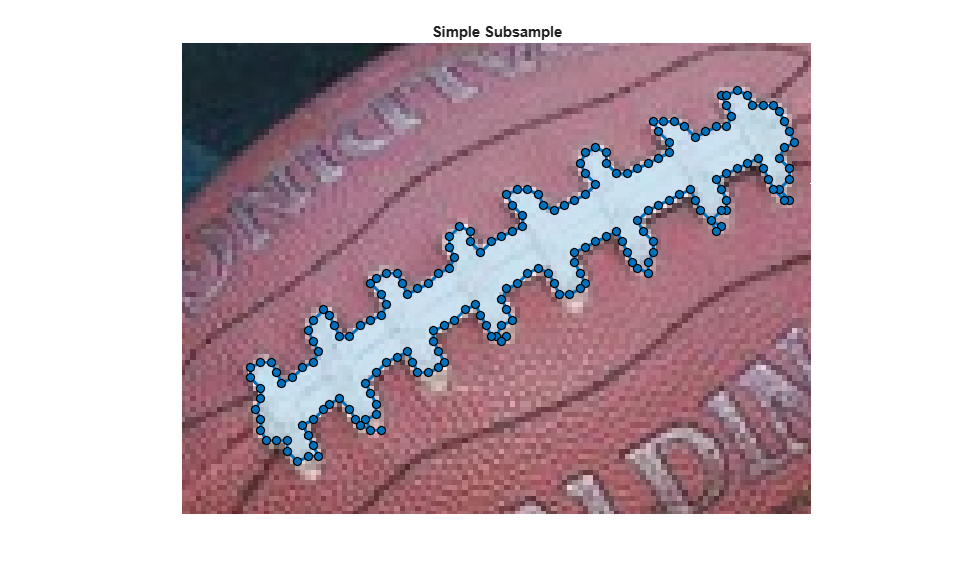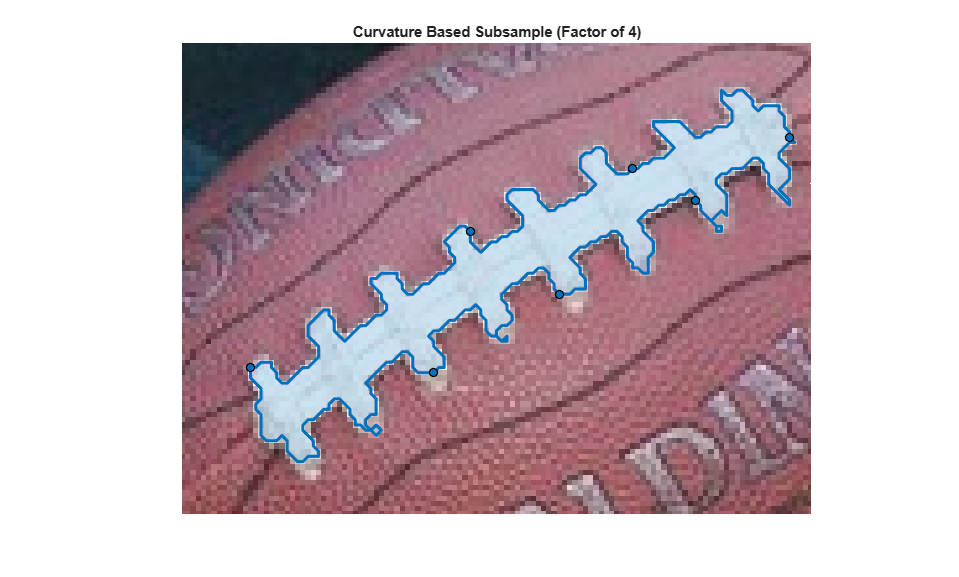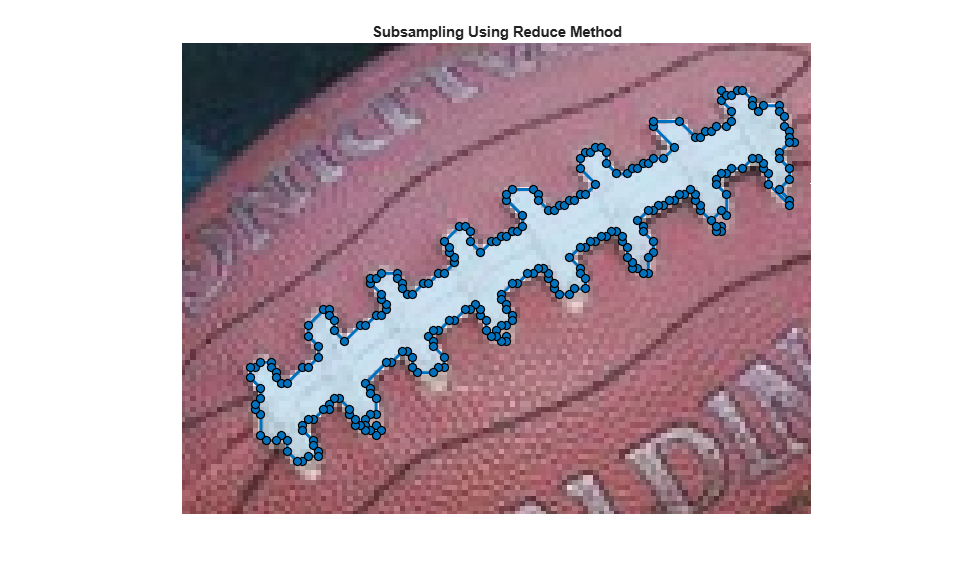# Subsample or Simplify a Freehand ROI

This example shows how to subsample or reduce the number of points in a `Freehand` ROI object.

### Introduction

The `drawfreehand` function creates a smooth-looking, freehand, region-of-interest (ROI). However, the edge of the ROI is actually made of discrete points distributed all along the boundary. Two factors contribute to how smooth a freehand ROI looks: 1) the density of points and 2) the `Smoothing` property of the freehand ROI object.

When drawing interactively, mouse motion determines the density of points. For large complex ROIs, the number of points used can be quite large.

The `Smoothing` property controls how the boundary looks. By default, the `Freehand` object uses a Gaussian smoothing kernel with a sigma value of 1 and a filter size of 5. Changing this value only changes how the boundary looks, it does not change the underlying `Position` property of the object.

### Default Density of Points

Reducing the density of points can help reduce the space required to store the ROI data and may also speed up any computation that depends on the number of these points. One way to reduce the density of points is to subsample the points, for example, pick every other point.

Create a sample freehand ROI by converting a mask to an ROI. The ROI is very dense since every boundary pixel will correspond to a point in the ROI.

```im = imread('football.jpg'); bw = im(:,:,1)>200; bw = bwareafilt(bw, 1); bloc = bwboundaries(bw,'noholes'); roipos = fliplr(bloc{1}); imshow(im); hfh = drawfreehand('Position', roipos); ```To visualize the density of the points, turn every point in the ROI into a waypoint.

```hfh.Waypoints(:) = true; title('Original density'); snapnow % Zoom in. xlim([80 200]); ylim([70 160]); snapnow ```### Subsampling the Position Points

Subsample the points that make up the `Position` property of the freehand ROI. Since the freehand ROI is very dense. Subsampling can substantially reduce the size without loosing fidelity. Query the initial, full, fine-grained position.

```fpos = hfh.Position; ```

Subsample, picking every other point.

```cpos = fpos(1:2:end,:); ```

Update the Position property of the ROI.

```hfh.Position = cpos; ```To see the density, turn all points into waypoints.

```hfh.Waypoints(:) = true; title('Simple Subsample'); snapnow ```### Subsampling - Using Rate of Change

A better approach to subsample the points would be to selectively start removing points which have low curvature. It makes more sense to remove a point that is along a relatively straight portion of the ROI rather than one near a curve. One simple approach to define a curvature value is to measure the rate of the change in position locations.

Measure the rate of change. The neighbor of the first point is the last point.

```dfpos = diff([fpos(end,:); fpos]); ```

Define an ad-hoc measure of curvature based on a simple low-pass filter.

```cm = sum(abs(conv2(dfpos, ones(3,2),'same')),2); ```

Sort by curvature.

```[~, cmInds] = sort(cm); ```

Pick 3/4 of the points with lower curvature values to remove from the ROI.

```numPointsToCull = round(0.25*size(fpos,1)); ```

Remove those positions.

```cpos = fpos; cpos(cmInds(1:numPointsToCull),:) = []; ```

Update the ROI, turning on all Waypoints to see the impact.

```hfh.Position = cpos; hfh.Waypoints(:) = true; title('Curvature Based Subsample (factor of 4)'); snapnow ```### Subsampling - Using `reduce` method on freehand ROI objects

An even better approach to subsample the points would be to use the `reduce` method on the ROI object. The `reduce` method operates directly on the `Position` property of the ROI object. You can affect the number of points removed by specifying a tolerance value between [0 1.0] as an optional input argument. The default value of tolerance is 0.01.

Reset the `Position` property and call `reduce` on the ROI object.

```hfh.Position = fpos; reduce(hfh); % View the updated ROI, turning all the points into waypoints to see the % impact. hfh.Waypoints(:) = true; title('Subsampling using reduce method'); snapnow ```### Interactive Subsampling

Another way to subsample is to use events to make this process easier. First create a listener to interactively change the number of points that the freehand ROI uses. Use the `UserData` property of the Freehand object to cache the full resolution `Position` data, along with the current value of tolerance. Then add a custom context menu to the ROI object by creating a new `uimenu` and parenting it to the `UIContextMenu` of the Freehand object. This menu option allows you to finalize the ROI, which deletes the temporary cache.

Restore the original ROI, and cache the original position along with its curvature measure in UserData.

```hfh.Waypoints(:) = true; hfh.UserData.fpos = fpos; hfh.UserData.tol = 0; ```Respond to mouse scroll.

```h = gcf; h.WindowScrollWheelFcn = @(h, evt) changeSampleDensity(hfh, evt); ```

Add a context menu to finalize the ROI and perform any clean up needed.

```uimenu(hfh.UIContextMenu, 'Text','Finalize',... 'MenuSelectedFcn', @(varargin)finalize(hfh)); title('Scroll to change density interactively'); ```### Animation of the Interactive Subsampling### Callback Function - Change the Sample Density Based on Mouse Scroll

This function gets called on scroll action. Scrolling up increases the density, and scrolling down decreases it. This allows you to interactively select the number of points to retain.

```function changeSampleDensity(hfh, evt) % Restore Position property of ROI. hfh.Position = hfh.UserData.fpos; % Change tolerance by a fixed amount based on the direction of the scroll. % This code changes the tolerance by 0.01 for every scroll increment. tol = hfh.UserData.tol + 0.01 * (evt.VerticalScrollCount); % Restrict the range of tolerance values to be from 0 to 0.15, which is the % useful range. tol = max(min(tol, 0.15), 0); % Call |reduce| with the specified tolerance. reduce(hfh,tol); hfh.UserData.tol = tol; % Update the ROI and turn all the points into waypoints to show the % density. hfh.Waypoints(:) = true; end ```

### Callback Function - Finalize the Freehand ROI

Delete and create a new Freehand ROI with the subsampled points to save on space.

```function finalize(hfh) h = ancestor(hfh, 'figure'); % Reset the mouse scroll wheel callback. h.WindowScrollWheelFcn = []; % Save finalized set of points. pos = hfh.Position; % Delete and create a new Freehand ROI with the new |Position| value. delete(hfh); drawfreehand(gca, 'Position', pos); end ```